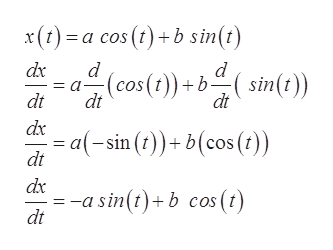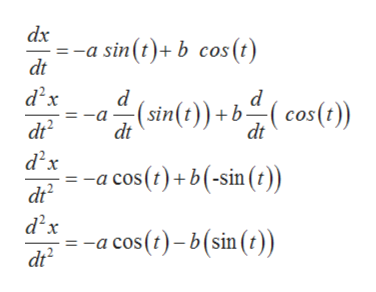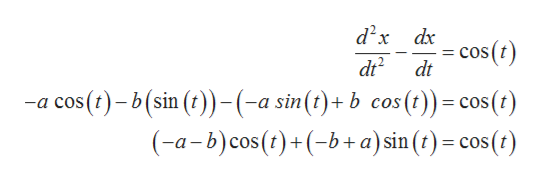Find constants a and b which make the function x(t)=acos(t)+bsin(t) a solution to the differential equation d2x/dt2 - dx/dt = cos(t)

Question

Find constants a and b which make the function x(t)=acos(t)+bsin(t) a solution to the differential equation d2x/dt- dx/dt = cos(t)

Step 1

If x(t)=a cos (t) + b sin(t) is a solution to the differential equation d2x/dt- dx/dt = cos(t) then x(t) = a cos(t) + b sin(t) satisfied the given differential equation.

So, differentiate x(t) = a cos(t) + b sin(t) with respect to the variable (t).help_outlineImage Transcriptionclosex)acos ()b sin(t) d dx (cos (t))bsint dt dt dt dx - a(-sin ())b(cos ()) dt dx a sin(t)+b cos (t) dt fullscreen
Step 2

Again, differentiate with respect to t:help_outlineImage Transcriptionclosedx =-a sin(t)+b cos (t) dt d2x (sin(t))bcos (t)) =-a dt2 dt dt d2x -a cos(t)+b(-sin ()) dt2 d2x -a cos (t)-b(sin ()) dt fullscreen
Step 3

Substitute the expression of dx/dt and d2x/dt2

In the equation d2x/dt2&...help_outlineImage Transcriptionclosed2x dx -cos() dt dt2 -a cos(t)-b(sin (t))-(-a sin(t)+b cos(t))= cos (t) (-a-b) cos (t)(ba) sin (t)= cos() COs fullscreen

Want to see the full answer?

See Solution

Want to see this answer and more?

Our solutions are written by experts, many with advanced degrees, and available 24/7

See Solution
Tagged in

Other